# Closures of Relations Epp section 10 CS 202

• Slides: 24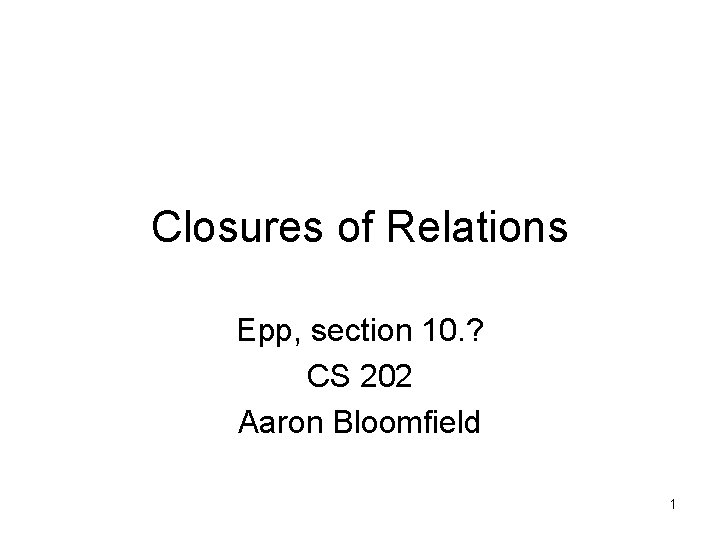Closures of Relations Epp, section 10. ? CS 202 Aaron Bloomfield 1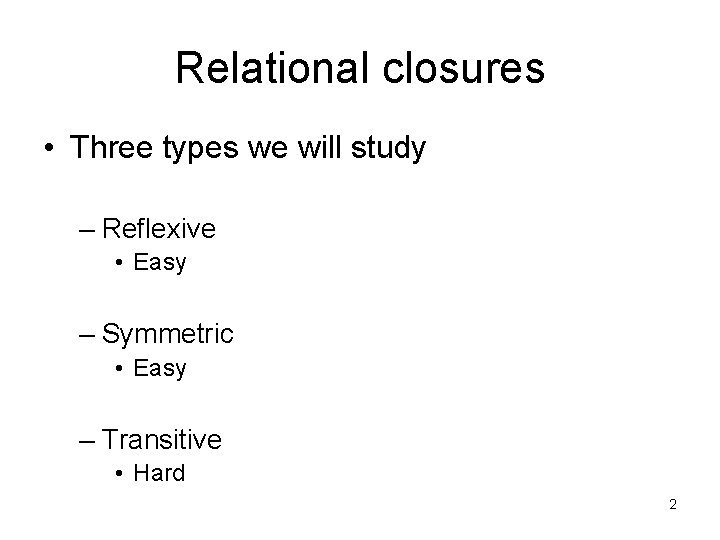Relational closures • Three types we will study – Reflexive • Easy – Symmetric • Easy – Transitive • Hard 2Reflexive closure • Consider a relation R: – From our Map. Quest example in the last slide set – Note that it is not reflexive • We want to add edges to make the relation reflexive • By adding those edges, we have made a nonreflexive relation R into a reflexive relation • This new relation is called the reflexive closure of R 3Reflexive closure • In order to find the reflexive closure of a relation R, we add a loop at each node that does not have one • The reflexive closure of R is R U – Where = { (a, a) | a R } • Called the “diagonal relation” – With matrices, we set the diagonal to all 1’s 4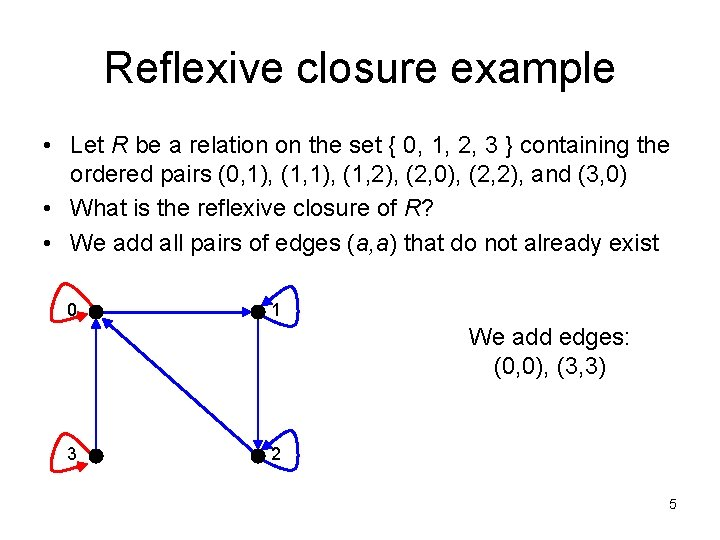Reflexive closure example • Let R be a relation on the set { 0, 1, 2, 3 } containing the ordered pairs (0, 1), (1, 2), (2, 0), (2, 2), and (3, 0) • What is the reflexive closure of R? • We add all pairs of edges (a, a) that do not already exist 0 1 We add edges: (0, 0), (3, 3) 3 2 5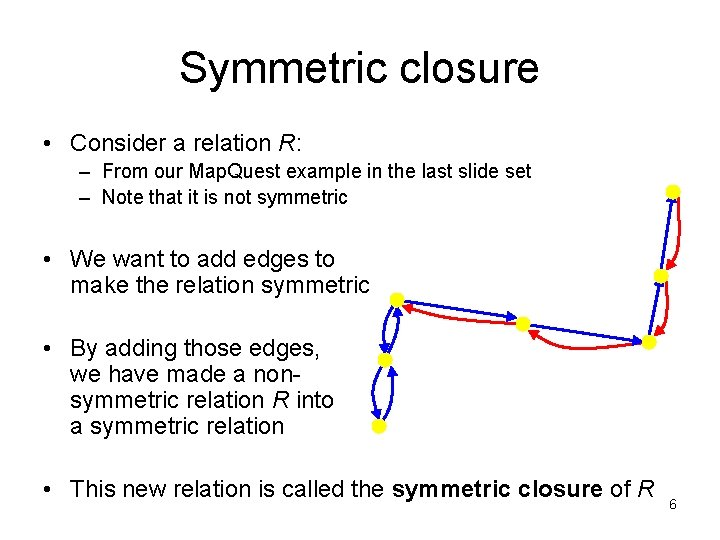Symmetric closure • Consider a relation R: – From our Map. Quest example in the last slide set – Note that it is not symmetric • We want to add edges to make the relation symmetric • By adding those edges, we have made a nonsymmetric relation R into a symmetric relation • This new relation is called the symmetric closure of R 6Symmetric closure • In order to find the symmetric closure of a relation R, we add an edge from a to b, where there is already an edge from b to a • The symmetric closure of R is R U R-1 – If R = { (a, b) | … } – Then R-1 = { (b, a) | … } 7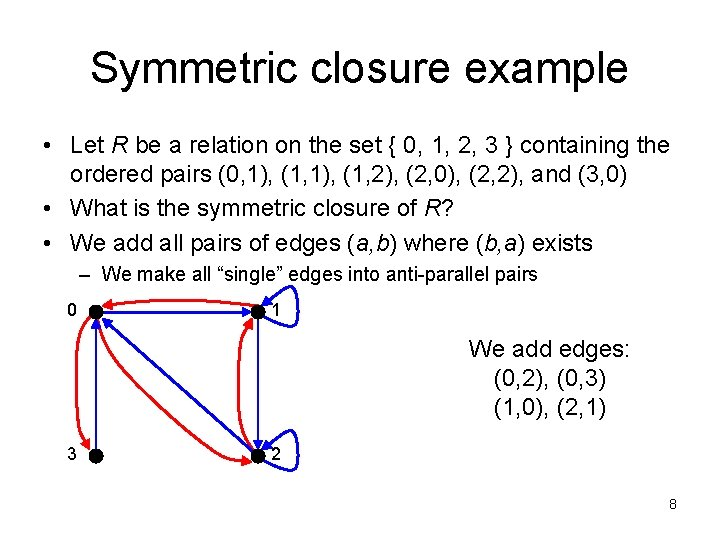Symmetric closure example • Let R be a relation on the set { 0, 1, 2, 3 } containing the ordered pairs (0, 1), (1, 2), (2, 0), (2, 2), and (3, 0) • What is the symmetric closure of R? • We add all pairs of edges (a, b) where (b, a) exists – We make all “single” edges into anti-parallel pairs 0 1 We add edges: (0, 2), (0, 3) (1, 0), (2, 1) 3 2 8Paths in directed graphs • A path is a sequences of connected edges from vertex a to vertex b • No path exists from the noted start location • A path that starts and ends at the same vertex is called a circuit or cycle Start (a) End (b) – Must have length ≥ 1 Start (a) 9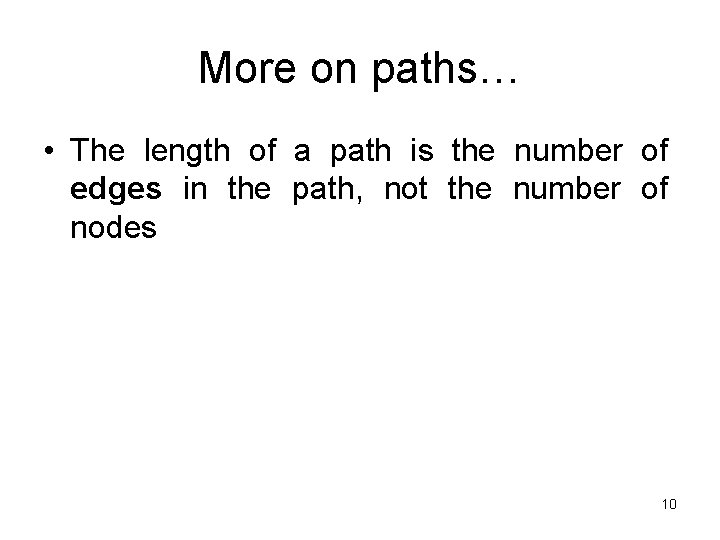More on paths… • The length of a path is the number of edges in the path, not the number of nodes 10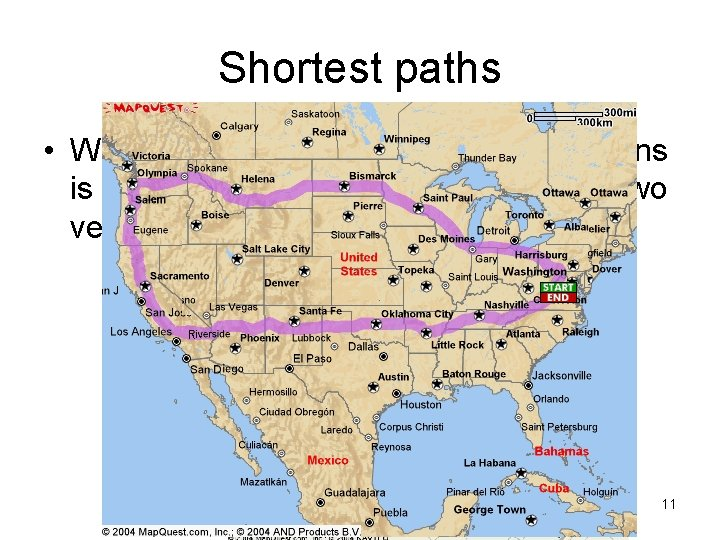Shortest paths • What is really needed in most applications is finding the shortest path between two vertices 11Transitive closure The transitive closure would contain edges between all nodes reachable by a path of any length 12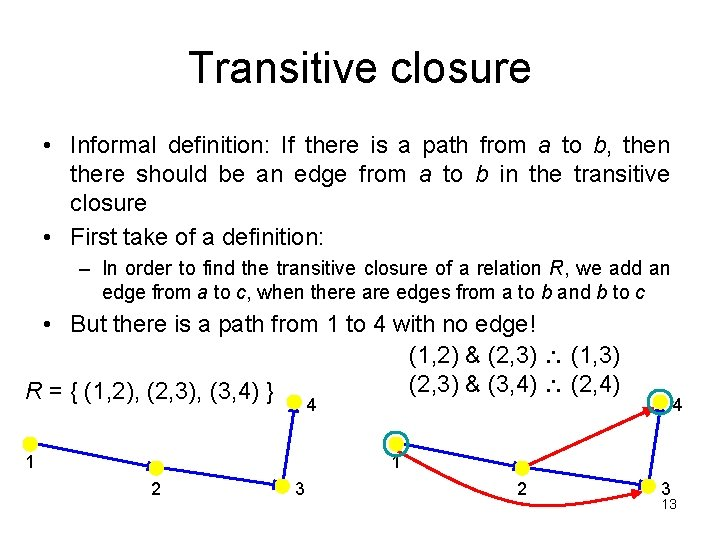Transitive closure • Informal definition: If there is a path from a to b, then there should be an edge from a to b in the transitive closure • First take of a definition: – In order to find the transitive closure of a relation R, we add an edge from a to c, when there are edges from a to b and b to c • But there is a path from 1 to 4 with no edge! (1, 2) & (2, 3) (1, 3) (2, 3) & (3, 4) (2, 4) R = { (1, 2), (2, 3), (3, 4) } 4 1 2 3 13Transitive closure • Informal definition: If there is a path from a to b, then there should be an edge from a to b in the transitive closure • Second take of a definition: – In order to find the transitive closure of a relation R, we add an edge from a to c, when there are edges from a to b and b to c – Repeat this step until no new edges are added to the relation • We will study different algorithms for determining the transitive closure • red means added on the first repeat • teal means added on the second repeat 4 1 2 3 146 degrees of separation • The idea that everybody in the world is connected by six degrees of separation – Where 1 degree of separation means you know (or have met) somebody else • Let R be a relation on the set of all people in the world – (a, b) R if person a has met person b • So six degrees of separation for any two people a and g means: – (a, b), (b, c), (c, d), (d, e), (e, f), (f, g) are all in R • Or, (a, g) R 6 15Connectivity relation • R contains edges between all the nodes reachable via 1 edge • R◦R = R 2 contains edges between nodes that are reachable via 2 edges in R • R 2◦R = R 3 contains edges between nodes that are reachable via 3 edges in R • Rn = contains edges between nodes that are reachable via n edges in R • R* contains edges between nodes that are reachable via any number of edges (i. e. via any path) in R – Rephrased: R* contains all the edges between nodes a and b when is a path of length at least 1 between a and b in R • R* is the transitive closure of R – The definition of a transitive closure is that there are edges between any nodes (a, b) that contain a path between them 16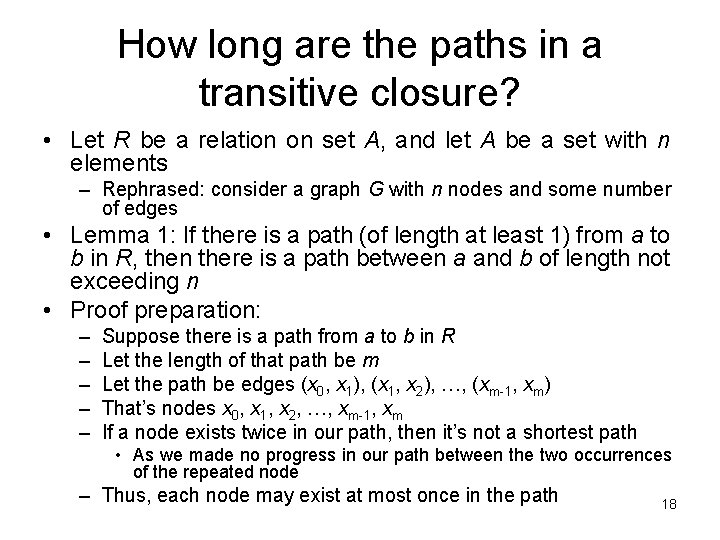How long are the paths in a transitive closure? • Let R be a relation on set A, and let A be a set with n elements – Rephrased: consider a graph G with n nodes and some number of edges • Lemma 1: If there is a path (of length at least 1) from a to b in R, then there is a path between a and b of length not exceeding n • Proof preparation: – – – Suppose there is a path from a to b in R Let the length of that path be m Let the path be edges (x 0, x 1), (x 1, x 2), …, (xm-1, xm) That’s nodes x 0, x 1, x 2, …, xm-1, xm If a node exists twice in our path, then it’s not a shortest path • As we made no progress in our path between the two occurrences of the repeated node – Thus, each node may exist at most once in the path 18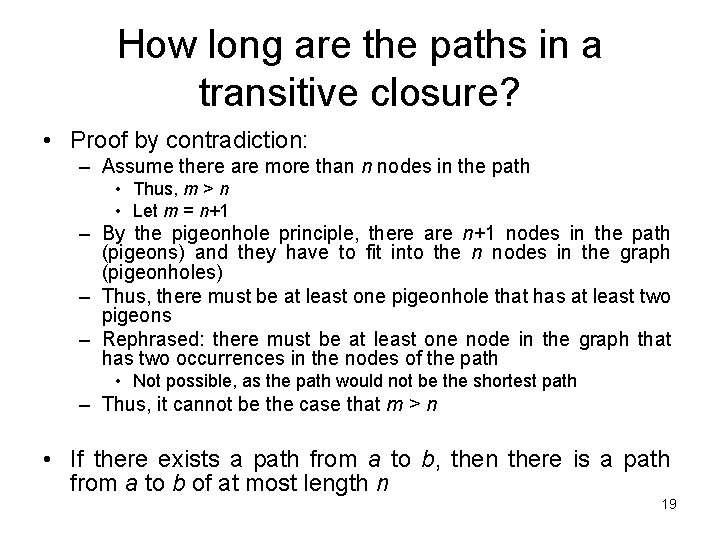How long are the paths in a transitive closure? • Proof by contradiction: – Assume there are more than n nodes in the path • Thus, m > n • Let m = n+1 – By the pigeonhole principle, there are n+1 nodes in the path (pigeons) and they have to fit into the n nodes in the graph (pigeonholes) – Thus, there must be at least one pigeonhole that has at least two pigeons – Rephrased: there must be at least one node in the graph that has two occurrences in the nodes of the path • Not possible, as the path would not be the shortest path – Thus, it cannot be the case that m > n • If there exists a path from a to b, then there is a path from a to b of at most length n 19Finding the transitive closure • Let MR be the zero-one matrix of the relation R on a set with n elements. Then the zero-one matrix of the transitive closure R* is: Nodes reachable with one application of the relation Nodes reachable with two applications of the relation Nodes reachable with n applications of the relation 20Sample questions • Find the zero-one matrix of the transitive closure of the relation R given by: 1 2 2 3 3 1 21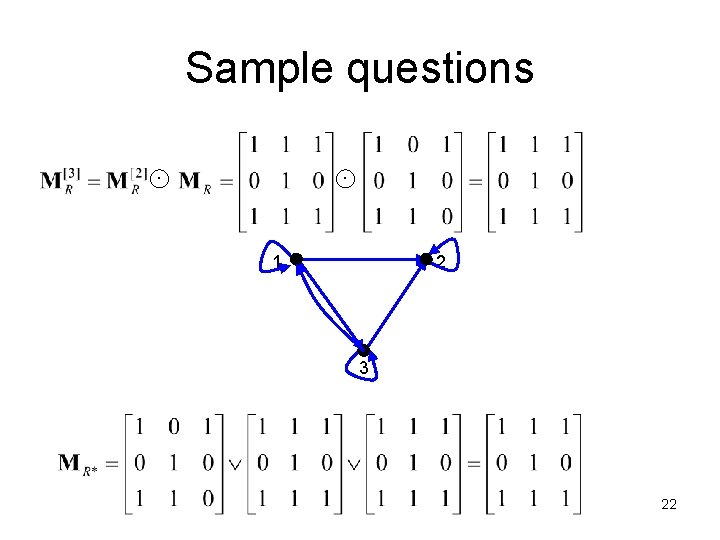Sample questions 1 2 3 22Transitive closure algorithm • What we did (or rather, could have done): – Compute the next matrix , where 1 ≤ i ≤ n – Do a Boolean join with the previously computed matrix • For our example: – Compute – Join that with to yield from above 23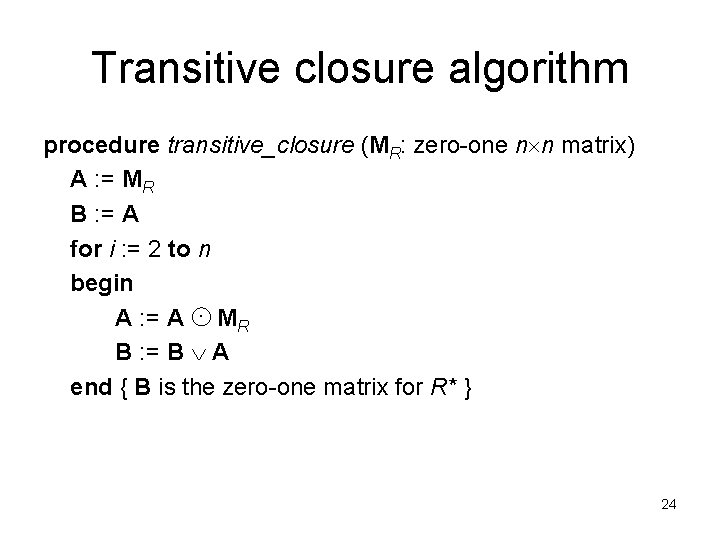Transitive closure algorithm procedure transitive_closure (MR: zero-one n n matrix) A : = MR B : = A for i : = 2 to n begin A : = A MR B : = B A end { B is the zero-one matrix for R* } 24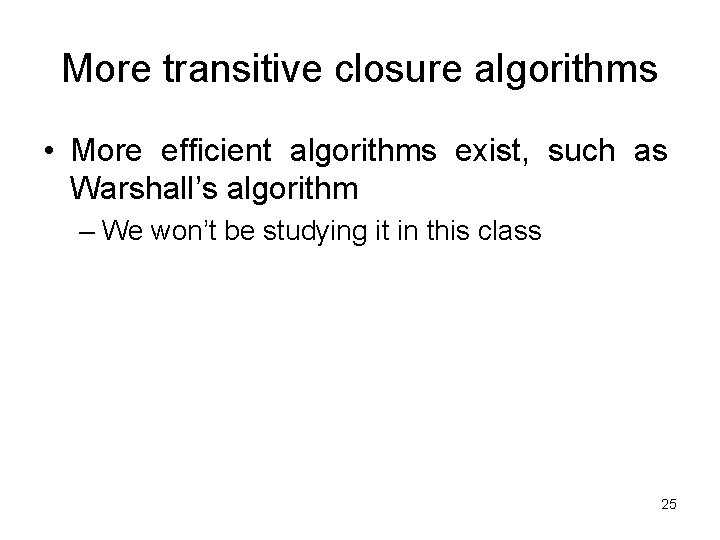More transitive closure algorithms • More efficient algorithms exist, such as Warshall’s algorithm – We won’t be studying it in this class 25